# Plots of spectral coefficients. [Graphical outputs.]

() More...

## Functions

void des_coef_xi (const Valeur &uu, int l, int k, int j, double pzero=1.e-14, const char *nomy=0x0, const char *title=0x0, const char *device=0x0, int newgraph=3, int nxpage=1, int nypage=1)
Plots the coefficients of the spectral expansion in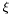of a Valeur .
void des_coef_theta (const Valeur &uu, int l, int k, int i, double pzero=1.e-14, const char *nomy=0x0, const char *title=0x0, const char *device=0x0, int newgraph=3, int nxpage=1, int nypage=1)
Plots the coefficients of the spectral expansion in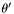of a Valeur .
void des_coef_phi (const Valeur &uu, int l, int j, int i, double pzero=1.e-14, const char *nomy=0x0, const char *title=0x0, const char *device=0x0, int newgraph=3, int nxpage=1, int nypage=1)
Plots the coefficients of the spectral expansion in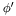of a Valeur .
void des_map_et (const Map_et &mp, int lz)
Plots the coefficients of the functions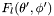and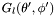of a mapping of class Map_et .

()

## Function Documentation

 void des_coef_phi ( const Valeur & uu, int l, int j, int i, double pzero = 1.e-14, const char * nomy = 0x0, const char * title = 0x0, const char * device = 0x0, int newgraph = 3, int nxpage = 1, int nypage = 1 )

Plots the coefficients of the spectral expansion inof a Valeur .

This routine performs a logarithmic plot of the coefficients of theexpansion of the coefficient which is in front of a givenbasis function (index j ) and a givenbasis function (index i ) in the general spectral expansion of a field. The plotted quantities are the logarithm of the absolute value of the coefficients, using solid lines (resp. dashed lines) for positive coefficients (resp. negative coefficients).

Parameters:
 uu [input] Valeur thecoefficients of which are to be plotted l [input] index of the domain j [input] index of the considered basis function ini [input] index of the considered basis function inpzero [input] positive number under which (in absolute value) a coefficient will be considered as zero (default value = 1.e-14) nomy [input] y legend of the figure (default value = 0x0, corresponds to no y legend) title [input] title of the figure (default value = 0x0, corresponds to no title) device [input] PGPLOT device (default value = 0x0) newgraph [input] controls the opening/closing of the graphic device: 0 : does nothing (the device must be already opened) 1 : opens the device but does not close it at the end 2 : closes the device at the end but does not open it at the beginning 3 (default value) : opens and closes the device nxpage [input] number of graphs in the horizontal direction of the display window (meaningfull only if newgraph = 1 or 3) (default value = 1) nypage [input] number of graphs in the vertical direction of the display window (meaningfull only if newgraph = 1 or 3) (default value = 1)
 void des_coef_theta ( const Valeur & uu, int l, int k, int i, double pzero = 1.e-14, const char * nomy = 0x0, const char * title = 0x0, const char * device = 0x0, int newgraph = 3, int nxpage = 1, int nypage = 1 )

Plots the coefficients of the spectral expansion inof a Valeur .

This routine performs a logarithmic plot of the coefficients of theexpansion of the coefficient which is in front of a givenbasis function (index k ) and a givenbasis function (index i ) in the general spectral expansion of a field. The plotted quantities are the logarithm of the absolute value of the coefficients, using solid lines (resp. dashed lines) for positive coefficients (resp. negative coefficients).

Parameters:
 uu [input] Valeur thecoefficients of which are to be plotted l [input] index of the domain k [input] index of the considered basis function ini [input] index of the considered basis function inpzero [input] positive number under which (in absolute value) a coefficient will be considered as zero (default value = 1.e-14) nomy [input] y legend of the figure (default value = 0x0, corresponds to no y legend) title [input] title of the figure (default value = 0x0, corresponds to no title) device [input] PGPLOT device (default value = 0x0) newgraph [input] controls the opening/closing of the graphic device: 0 : does nothing (the device must be already opened) 1 : opens the device but does not close it at the end 2 : closes the device at the end but does not open it at the beginning 3 (default value) : opens and closes the device nxpage [input] number of graphs in the horizontal direction of the display window (meaningfull only if newgraph = 1 or 3) (default value = 1) nypage [input] number of graphs in the vertical direction of the display window (meaningfull only if newgraph = 1 or 3) (default value = 1)
 void des_coef_xi ( const Valeur & uu, int l, int k, int j, double pzero = 1.e-14, const char * nomy = 0x0, const char * title = 0x0, const char * device = 0x0, int newgraph = 3, int nxpage = 1, int nypage = 1 )

Plots the coefficients of the spectral expansion inof a Valeur .

This routine performs a logarithmic plot of the coefficients of theexpansion of the coefficient which is in front of a givenbasis function (index k ) and a givenbasis function (index j ) in the general spectral expansion of a field. The plotted quantities are the logarithm of the absolute value of the coefficients, using solid lines (resp. dashed lines) for positive coefficients (resp. negative coefficients).

Parameters:
 uu [input] Valeur thecoefficients of which are to be plotted l [input] index of the domain k [input] index of the considered basis function inj [input] index of the considered basis function inpzero [input] positive number under which (in absolute value) a coefficient will be considered as zero (default value = 1.e-14) nomy [input] y legend of the figure (default value = 0x0, corresponds to no y legend) title [input] title of the figure (default value = 0x0, corresponds to no title) device [input] PGPLOT device (default value = 0x0) newgraph [input] controls the opening/closing of the graphic device: 0 : does nothing (the device must be already opened) 1 : opens the device but does not close it at the end 2 : closes the device at the end but does not open it at the beginning 3 (default value) : opens and closes the device nxpage [input] number of graphs in the horizontal direction of the display window (meaningfull only if newgraph = 1 or 3) (default value = 1) nypage [input] number of graphs in the vertical direction of the display window (meaningfull only if newgraph = 1 or 3) (default value = 1)
 void des_map_et ( const Map_et & mp, int lz )

Plots the coefficients of the functionsandof a mapping of class Map_et .

This routine performs a logarithmic plot of the coefficients of the(resp.) expansion of the coefficient which is in front of a given(resp.) basis function (index k (resp. index j )). The plotted quantities are the logarithm of the absolute value of the coefficients, using solid lines (resp. dashed lines) for positive coefficients (resp. negative coefficients).

Parameters:
 mp [input] Mapping of class Map_et lz [input] Index of the domain where the plot is to performed

Generated on 7 Dec 2019 for LORENE by1.6.1